# AVL Tree, Left and right rotations

Learn: In this article, we are going to learn what is AVL tree? And how to use left and right rotations in an AVL tree?
Submitted by Amit Shukla, on July 23, 2017

### AVL Tree - Definition

In early 60’s of 19th century E.M. Landis and G.M. Adelson- Velsky formed a self - balancing BST (binary search tree) data structure. This data structure is known by AVL tree.

An AVL tree is a binary search tree with self – balancing condition. The condition assures that the difference between the height of left and right sub tree cannot be greater than one. This difference between left sub tree and right sub tree is known as Balance Factor.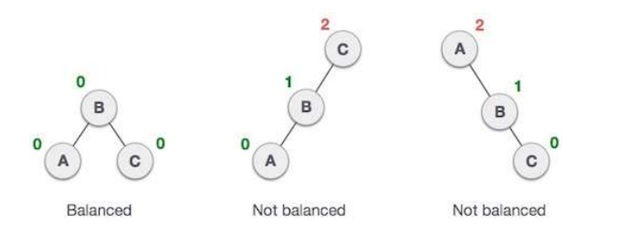In the second tree, the left subtree of C has height 2 and the right subtree has height 0, so the difference is 2. In the third tree, the right subtree of A has height 2 and the left is missing, so it is 0, and the difference is 2 again. AVL tree permits difference (balance factor) to be only 1.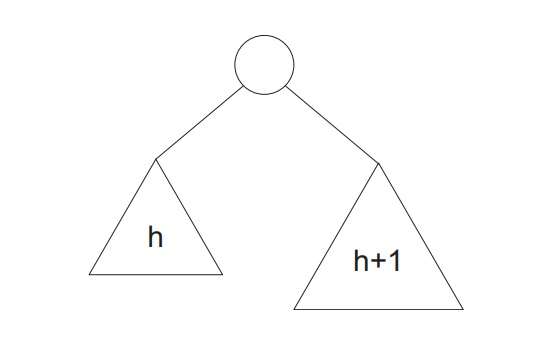In the above figure we can observe that the difference of height of left subtree and height of right subtree is 1, hence we can call it as an AVL tree.

Balance factor can be calculated by subtracting height of left subtree from height of right subtree.

BALANCE FACTOR = HEIGHT OF LEFT SUBTREE – HEIGHT OF RIGHT SUBTREE

If the value of balance factor is greater than one then the tree is balanced using some rotational techniques and these rotational techniques are known as AVL rotation.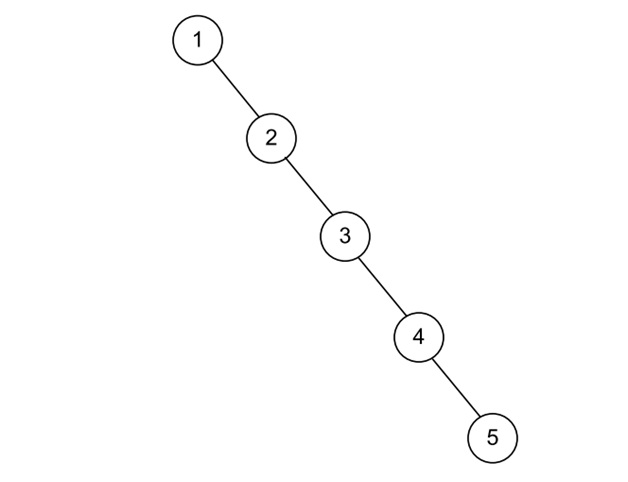In above figure we can see that the 5 elements were not in form of AVL balance tree because the height of left subtree is 5 and height of right subtree is 0.

According to formula the balance factor of this tree is 5 hence it’s not an AVL balance tree.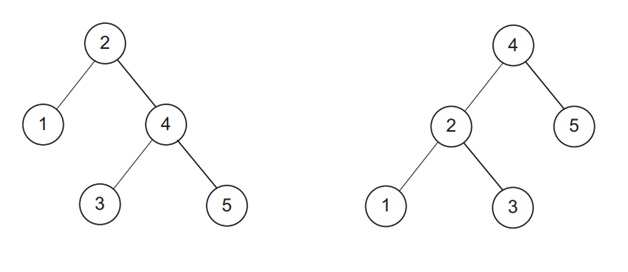In above figure we can see that in both cases the 5 elements are arranged in an AVL balance tree. Because in both cases the value of balance factor is 1.

## AVL ROATATIONS

AVL tree have following rotation techniques to balance itself.

1. Left rotation
2. Right rotation
3. Left - Right rotation
4. Right - Left rotation

In above techniques the first two are single rotations and next two are double rotations.

### Left Rotation

If the node is inserted into the right subtree of the right subtree then we can perform the left rotation to balance the unbalanced tree.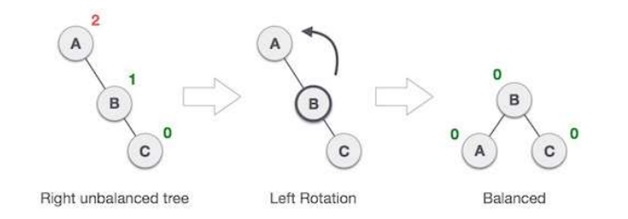In above figure we can see the left rotation of the tree. Using this technique we balance an unbalance tree of height 2.

### Right Rotation

If the node is inserted into the left subtree of the leftsubtree then we can perform the right rotation to balance the unbalanced tree.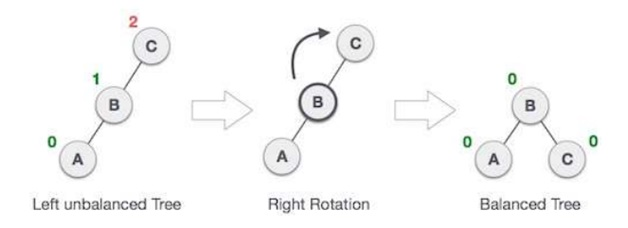In above figure we can see the right rotation of the tree. Using this technique we balance an unbalance tree of height 2.

Image Reference:

Top MCQs